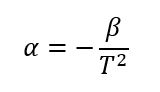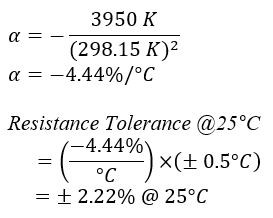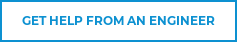# NTC Thermistor Calculating the Temperature Coefficient of a Thermistor

The temperature coefficient of a thermistor is defined as the relative change in resistance referred to the change in temperature. The easiest way to calculate the temperature coefficient is by using the below equation:Temperature Coefficient Equation

Where:

(For more information and to learn to calculate Beta, visit our page NTC Thermistor Beta or check our our blog on The Secret to Successful Thermistor Beta Calculations.)

The temperature coefficient of a thermistor is used when calculating the tolerance of a thermistor in terms of temperature. This unit is measured in terms of the percent per degrees Celsius (%/°C).

(EX): Suppose a customer orders a 10,000 W thermistor with an accuracy of ± 0.5°C.

(Q) How would we translate this in terms of resistance tolerance at different temperatures?

(A) The specified part number would be NT03 10391, with a beta of 3950 K. The tolerance at 25°C (298.15 K) would be:Temperature Coefficient Equation Example

In this manner, you can find the tolerance at any temperature for any given value of beta. Also, the reverse is true if you need to find the accuracy of a part in terms of temperature.